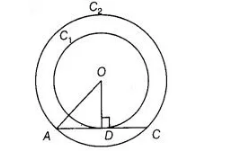# Out of the two concentric circles,

Question:

Out of the two concentric circles, the radius of the outer circle is 5 cm and the chord AC of length 8 cm is a tangent to the inner circle. Find the radius of

the inner circle.

Solution:

Let Cand C2 be the two circles having same centre O. AC is a chord which touches the C1 at point D.Join $O D$.

Also, $O D \perp A C$

$\therefore \quad A D=D C=4 \mathrm{~cm} \quad$ [perpendicular line OD bisects the chord]

In right angled $\triangle A O D, \quad O A^{2}=A D^{2}+D O^{2}$

[by Pythagoras theorem, i.e., (hypotenuse) $^{2}=(\text { base })^{2}+$ (perpendicular) $^{2}$ ]

$\Rightarrow \quad D O^{2}=5^{2}-4^{2}$

$=25-16=9$

$\Rightarrow \quad D O=3 \mathrm{~cm}$

$\therefore$ Radius of the inner circle $O D=3 \mathrm{~cm}$